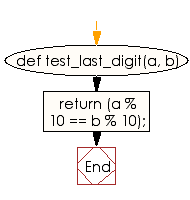﻿ Ruby Basic exercises: Check two non-negative integer values and return true if they have the same last digit - w3resource# Ruby Basic Exercises: Check two non-negative integer values and return true if they have the same last digit

## Ruby Basic: Exercise-30 with Solution

Write a Ruby program to check two non-negative integer values and return true if they have the same last digit.

Sample array : ["Ruby", 2.3, Time.now]

Ruby Code:

``````def test_last_digit(a, b)
return (a % 10 == b % 10);
end
print test_last_digit(5, 5),"\n"
print test_last_digit(15, 25),"\n"
print test_last_digit(256, 346),"\n"
print test_last_digit(26, 34)
``````

Output:

```true
true
true
false
```

Flowchart:Ruby Code Editor: